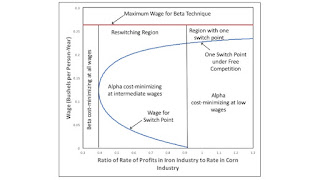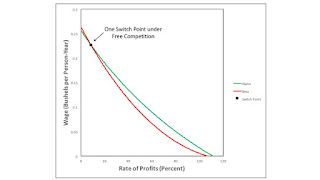## Saturday, March 18, 2017

### Reswitching Only Under OligopolyFigure 1: Rates of Profits for Switch Points for Differential Rates of Profits
1.0 Introduction

Suppose one knows the technology available to firms at a given point in time. That is, one knows the techniques among which managers of firms choose. And suppose one finds that reswitching cannot occur under this technology, given prices of production in which the same rate of profits prevails among all industries. But, perhaps, barriers to entry persist. If one analyzes the choice of technique for the given technology, under the assumption that prices of production reflect stable (non-unit) ratios of profits, differing among industries, reswitching may arise for the technology. The numerical example in this post demonstrates this logical possibility.

The numerical example follows a model of oligopoly I have previously outlined. In some sense, the example is symmetrical to the example in this draft paper. That example is of a reswitching example under pure competition, which becomes an example without reswitching and capital reversing, if the ratio of the rates of profits among industries differs enough. The example in this post, on the other hand, has no reswitching or capital reversing under pure competition. But if the ratios of the rates of profits becomes extreme enough, it becomes a reswitching example.

2.0 Technology

The technology for this example resembles many I have explained in past posts. Suppose two commodities, iron and corn, are produced in the example economy. As shown in Table 1, two processes are known for producing iron, and one corn-producing process is known. Each column lists the inputs, in physical units, needed to produce one physical unit of the output for the industry for that column. All processes exhibit Constant Returns to Scale, and all processes require services of inputs over a year to produce output of a single commodity available at the end of the year. This is an example of a model of circulating capital. Nothing remains at the end of the year of the capital goods whose services are used by firms during the production processes.

 Input IronIndustry CornIndustry Labor 1 305/494 1 Iron 1/10 229/494 11/10 Corn 1/40 3/1976 2/5

For the economy to be self-reproducing, both iron and corn must be produced each year. Two techniques of production are available. The Alpha technique consists of the first iron-producing process and the lone corn-producing process. The Beta technique consists of the remaining iron-producing process and the corn-producing process.

3.0 Price Equations

The choice of technique is analyzed on the basis of cost-minimization, with prices of production. Suppose the Alpha technique is cost minimizing. Then the following system of equalities and inequalities hold:

[(1/10)p + (1/40)](1 + rs1) + w = p
[(229/494)p + (3/1976)](1 + rs1) + (305/494)wp
[(11/10)p + (2/5)](1 + rs2) + w = 1

where p is the price of a unit of iron, and w is the wage.

The parameters s1 and s2 are given constants, such that rs1 is the rate of profits in iron production and rs2 is the rate of profits in corn production. The quotient s1/s2 is the ratio, in this model, of the rate of profits in iron production to the rate of profits in corn production. Consider the special case:

s1 = s2 = 1

This is the case of free competition, with investors having no preference among industries. In this case, r is the rate of profits. I call r the scale factor for the rate of profits in the general case where s1 and s2 are unequal.

The above system of equations and inequalities embody the assumption that a unit corn is the numeraire. They also show labor as being advanced and wages as paid out of the surplus at the end of the period of production. If the second inequality is an equality, both the Alpha and the Beta techniques are cost-minimizing; this is a switch point. The Alpha technique is the unique cost-minimizing technique if it is a strict inequality. To create a system expressing that the Beta technique is cost-minimizing, the equality and inequality for iron production are interchanged.

4.0 Choice of Technique

The above system can be solved, given s1, s2, and the scale factor for the rate of profits. I record the solution for a couple of special cases, for completeness. Graphs of wage curves and a bifurcation diagram illustrate that stable (non-unitary) ratios of rates of profits can change the dynamics of markets.

4.1 Free Competition

Consider the special case of free competition. The wage curve for the Alpha technique is:

wα = (41 - 38r + r2)/[80(2 + r)]

The price of iron, when the Alpha technique is cost-minimizing, is:

pα = (5 - 3r)/[8(2 + r)]

The wage curve for the Beta technique is:

wβ = (6,327 - 9,802r + 3,631r2)/[20(1,201 + 213r)]

When the Beta technique is cost-minimizing, the price of iron is:

pβ = [5(147 - 97r)]/[2(1,201 + 213r)]

Figure 2 graphs the wage curves for the two techniques, under free competition and a uniform rate of profits among industries. The wage curves intersect at a single switch point, at a rate of profits of, approximately, 8.4%:

rswitch = (1/1,301)[799 - 24 (8261/2)]

The wage curve for the Beta technique is on the outer envelope, of the wage curves, for rates of profits below the switch point. Thus, the Beta technique is cost-minimizing for low rates of profits. The Alpha technique is cost minimizing for feasible rates of profits above the switch point. Around the switch point, a higher rate of profits is associated with the adoption of a less capital-intensive technique. Under free competition, this is not a case of capital-reversing.Figure 2: Wage Curves for Free Competition

4.2 A Case of Oligopoly

Now, I want to consider a case of oligopoly, in which firms in different industries are able to ensure long-lasting barriers to entry. These barriers manifest themselves with the following parameter values:

s1 = 4/5
s2 = 5/4

In this case, the wage curve for the Alpha technique is:

wα = (4,100 - 4,435r + 100r2)/[40(400 + 259r)]

The price of iron, when the Alpha technique is cost-minimizing, is:

pα = (125 - 96r)/(400 + 259r)

The wage curve for the Beta technique is:

wβ = 8(126,540 - 195,289r + 72,620r2)/[160(24,020 + 9,447r)]

The price of iron, when the Beta technique is cost-minimizing, is:

pβ = 2(3,675 - 3,038r)/(24,020 + 9,447r)

Figure 3 graphs the wage curves for the Alpha and Beta techniques, for the parameter values for this model of oligopoly. This is now an example of reswitching. The Beta technique is cost minimizing at low and high rates of profits. The Alpha technique is cost minimizing at intermediate rates. The switch points are at, approximately, a value of the scale factor for rates of profits of 12.07% and 77.66%, respectively.Figure 3: Wage Curves for a Case of Oligopoly

4.3 A Range of Ratios of Profit Rates

The above example of oligopoly can be generalized. I restrict myself to the case where the parameters expressing the ratio of rates of profits between industries satisfy:

s2 = 1/s1

One can then consider how the shapes and locations of wage curves and switch points vary with continuous variation in s1/s2. Figure 1, at the top of this post, graphs the wage at switch points for a range of ratios of rates of profits. Since the Beta technique is cost-minimizing, in the graph, at all high feasible wages and low scale factor for the rates of profits, I only graph the maximum wage for the Beta technique. I do not graph the maximum wage for the Alpha technique.

As the ratio of the rate of profits in the iron industry to rate in the corn industry increases towards unity, the model changes from a region in which the Beta technique is dominant to a reswitching example to an example with only a single switch point. As expected, only one switch point exists when the rate of profits is uniform between industries.

5.0 Conclusion

So I have created and worked through an example where:

• No reswitching or capital-reversing exists under pure competition, with all industries earning the same rate of profits.
• Reswitching and capital-reversing can arise for oligopoly, with persistent differential rates of profits across industries.

No qualitative difference necessarily exists, in the long period theory of prices, between free competition and imperfections of competition. Doubtless, all sorts of complications of strategic behavior, asymmetric information, and so on are empirically important. But it seems confused to blame the failure of markets to clear or economic instability on such imperfections.